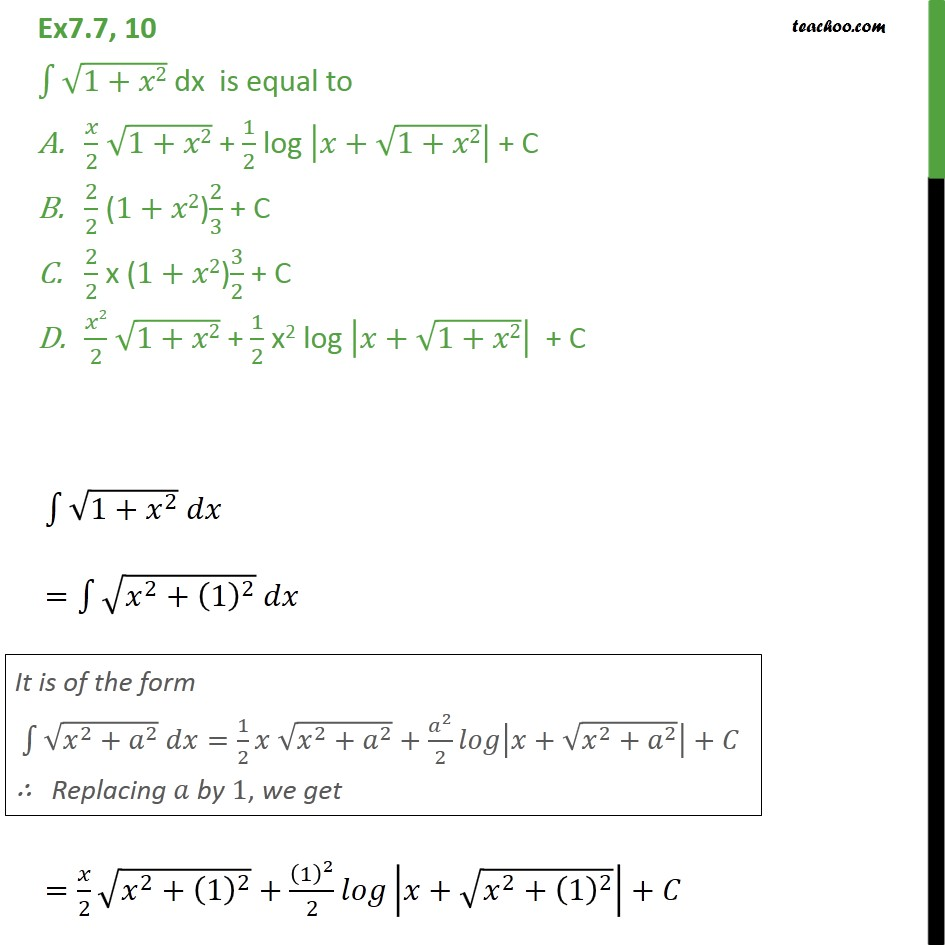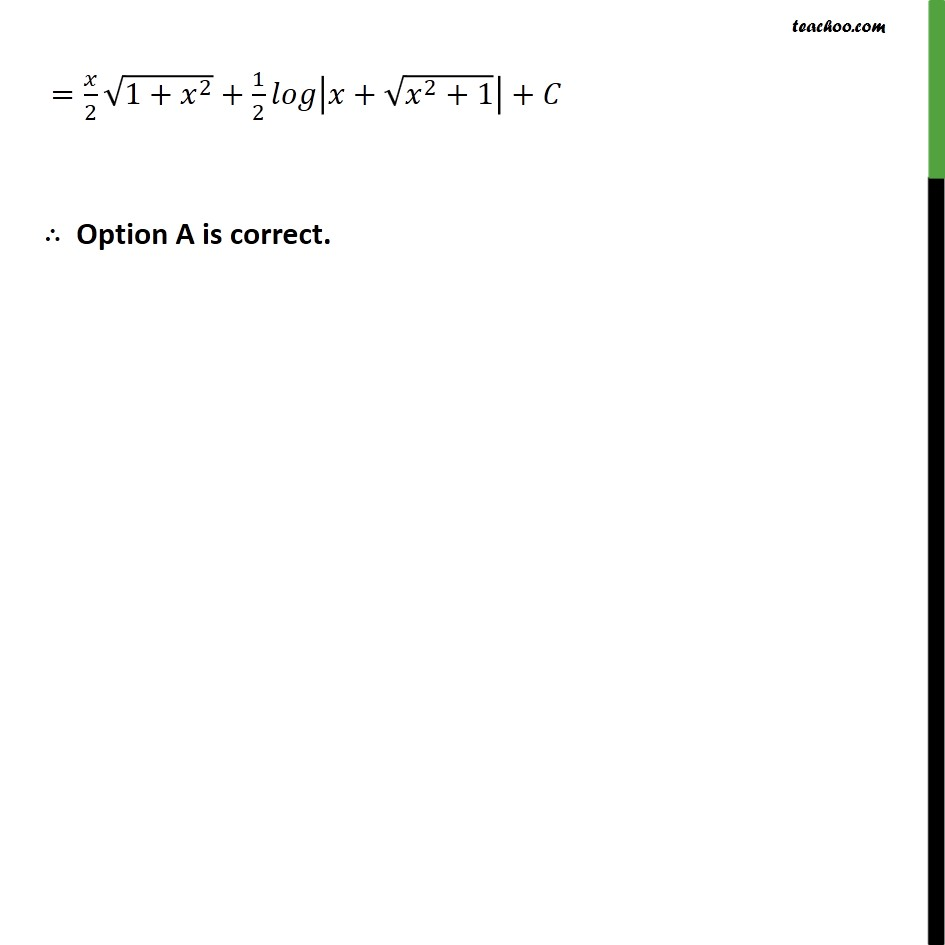Ex 7.7

Chapter 7 Class 12 Integrals
Serial order wiseLearn in your speed, with individual attention - Teachoo Maths 1-on-1 Class

### Transcript

Ex7.7, 10 1+ 2 dx is equal to 2 1+ 2 + 1 2 log + 1+ 2 + C 2 2 (1+ 2) 2 3 + C 2 2 x (1+ 2) 3 2 + C 2 2 1+ 2 + 1 2 x2 log + 1+ 2 + C 1+ 2 = 2 + 1 2 = 2 2 + 1 2 + 1 2 2 + 2 + 1 2 + = 2 1+ 2 + 1 2 + 2 +1 + Option A is correct.